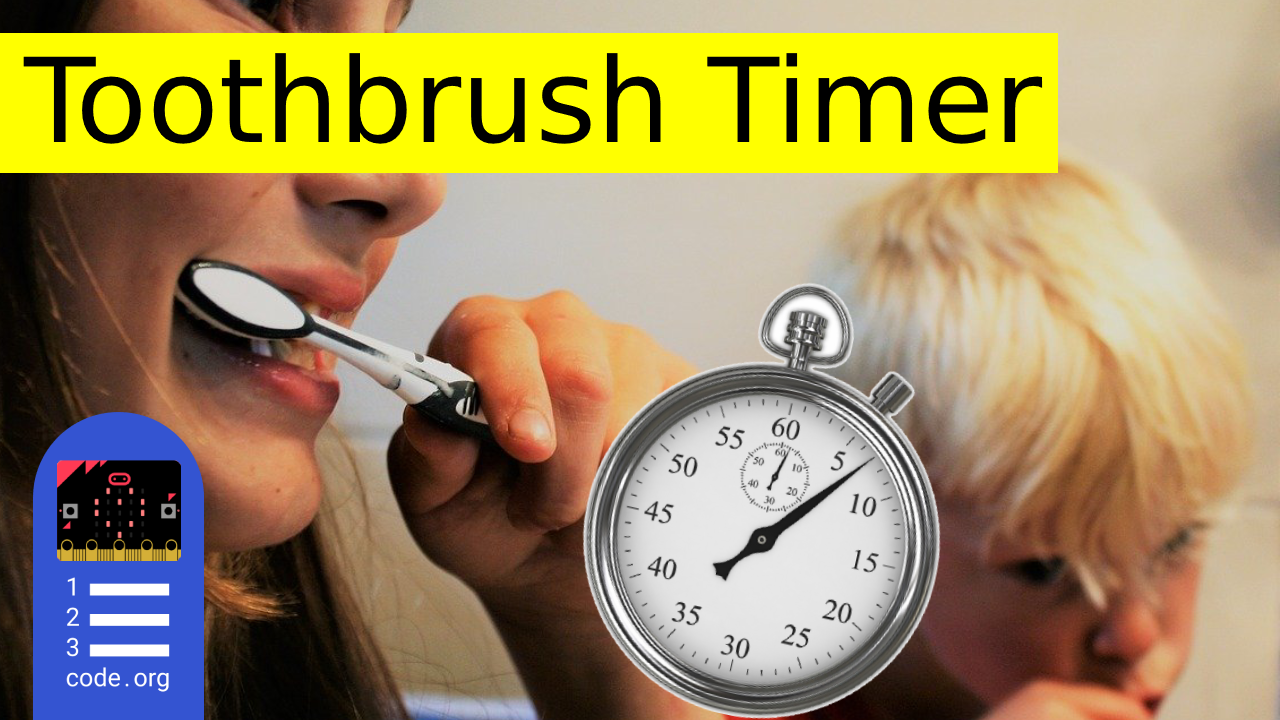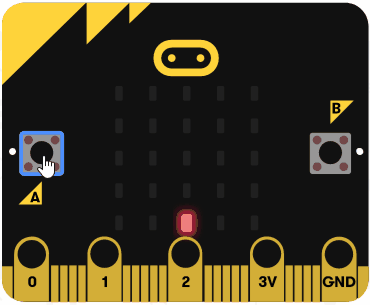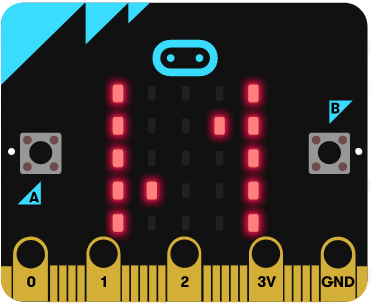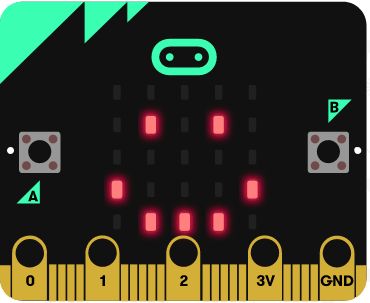# Microbit Toothbrush Timer

## Mathematics MicrobitNormal

In this project you will turn your microbit into a toothbrush timer! Dentists recommend that you brush your teeth for 2 minutes so we will program our microbits to count 2 minutes and tell us how much time has elapsed and when to switch from your top teeth to your bottom teeth!1

2

#### Create a 'seconds' variable

We're going to count to 2 minutes (or 120 seconds) so we need a variable to store the seconds as they tick by.

Create a variable called 'seconds'.

3

#### Display an arrow

To start the toothbrush timer you will need to press the A button. So we will show an arrow pointing left (west) at the start. This will point at the A button.

You will find these blocks in the Advanced > Images toolbox.

Note: Change the arrow image to West (North is displayed in the code below).

```images.arrowImage(ArrowNames.West).showImage(0)

```4

#### Count to 120 seconds

Next we will program the A button to set the 'seconds' variable to 0 and then count to 120.

We do this by using a 'while' loop block. While the 'seconds' variable is less than or equal to 120, pause 1 second and then add 1 to the 'seconds' variable. This will keep adding 1 to the 'seconds' variable until it reaches 120.

```input.onButtonPressed(Button.A, function () {
seconds = 0
while (seconds <= 120) {
basic.pause(1000)
seconds += 1
}
})

```

5

#### Add an 'if then else'

We're going to check how many seconds have passed and display a graph using the LEDs to show how many have.

To do this we first need to add an 'if then else' block inside the 'forever' block. We then use the plus button on the 'if then else' block to set it up like below:

```basic.forever(function () {
if (true) {

} else if (false) {

} else if (false) {

} else if (false) {

}
})

```

6

#### Setup the conditions

Now we will setup the conditions in the 'if then else' block.

This is what we want to test and what should happen:

 Condition(s) What should happen seconds greater than 0 AND less than 60 show a bar graph of the amount of seconds that have passed (between 0 and 60) seconds equals 60 show an image to let you know to switch from your top teeth to your bottom (or vice versa!) seconds greater than 60 AND less than 120 show a bar graph of the amount of seconds that have passed (between 60 and 120) seconds equals 120 show a smiley face as you've finished

```basic.forever(function () {
if (seconds > 0 && seconds < 60) {

} else if (seconds == 60) {

} else if (seconds > 60 && seconds < 120) {

} else if (seconds == 120) {

}
})

```

7

#### Show a graph for the 1st minute

Using the Led toolbox, add the following code into the 'if then else' block.

This will show a bar graph depending how many seconds have passed between 0 and 60.

```basic.forever(function () {
if (seconds > 0 && seconds < 60) {
led.plotBarGraph(
seconds,
60
)
} else if (seconds == 60) {

} else if (seconds > 60 && seconds < 120) {

} else if (seconds == 120) {

}
})

```8

#### Show a switch image

When you get to 1 minute (or 60 seconds), show an image to let you know to switch from top to bottom (or vice versa!).

Add a 'show leds' block and draw an image the symbolises switching.

```basic.forever(function () {
if (seconds > 0 && seconds < 60) {
led.plotBarGraph(
seconds,
60
)
} else if (seconds == 60) {
basic.showLeds(`
# . . . #
# . . # #
# . . . #
# # . . #
# . . . #
`)
} else if (seconds > 60 && seconds < 120) {

} else if (seconds == 120) {

}
})

```9

#### Show a graph for the 2nd minute

Now for the 2nd minute we will again show a bar graph but this time as the 'seconds' variable is counting between 60 and 120, we need to subtract 60 from the 'seconds' variable when we pass it into the 'plot bar graph of' block.

```basic.forever(function () {
if (seconds > 0 && seconds < 60) {
led.plotBarGraph(
seconds,
60
)
} else if (seconds == 60) {
basic.showLeds(`
# . . . #
# . . # #
# . . . #
# # . . #
# . . . #
`)
} else if (seconds > 60 && seconds < 120) {
led.plotBarGraph(
seconds - 60,
60
)
} else if (seconds == 120) {

}
})

```10

#### Show a smiley face when done

Finally, show a smiley face icon once seconds equals 120 and you're done.

```basic.forever(function () {
if (seconds > 0 && seconds < 60) {
led.plotBarGraph(
seconds,
60
)
} else if (seconds == 60) {
basic.showLeds(`
# . . . #
# . . # #
# . . . #
# # . . #
# . . . #
`)
} else if (seconds > 60 && seconds < 120) {
led.plotBarGraph(
seconds - 60,
60
)
} else if (seconds == 120) {
basic.showIcon(IconNames.Happy)
}
})
```End of lesson